# CRYPT-5 消息认证和哈希函数

author : Odyssey@cyberpeace

### 消息认证

• 根据消息和秘密密钥生成一个短序列位
• 为了验证消息，通信双方将计算相同的位模式，假设他共享相同的密钥

• 消息可以以纯文本形式发送，并附加验证码
• 这不是数字签名：接收者可以生成相同的MAC
• 可以使用他的私钥对验证者进行加密以产生 数字签名
• 可以加密消息和认证者

• 内容修改
• 序列修改：修改一系列消息，包括插入，删除，重新排序
• 定时修改：延迟或重播消息

### 5.2.1 消息认证码的定义及使用方式

• 接收方相信发送方发来的消息未被篡改，这是因为攻击者不知道密钥，所以不能够在篡改消息后相应地篡改MAC，而如果仅篡改消息，则接收方计算的新MAC将与收到的MAC不同。
• 接收方相信发送方不是冒充的，这是因为除收发双方外再无其他人知道密钥，因此其他人不可能对自己发送的消息计算出正确的MAC。

#### 5.2.1 消息认证码的定义及使用方式

AC函数与加密算法类似，不同之处为MAC函数不必是可逆的，因此与加密算法相比更不易被攻破。 上述过程中，由于消息本身在发送过程中是明文形式，所以这一过程只提供认证性而未提供保密性。为提供保密性可在MAC函数以后(如图5.1(b))或以前(如图5.1(c))进行一次加密，而且加密密钥也需被收发双方共享。在图5.1(b)中，M与MAC链接后再被整体加密，在图5.1(c)中，M先被加密再与MAC链接后发送。通常希望直接对明文进行认证，因此图5.1(b)所示的使用方式更为常用。

#### 5.2.1 消息认证码的定义及使用方式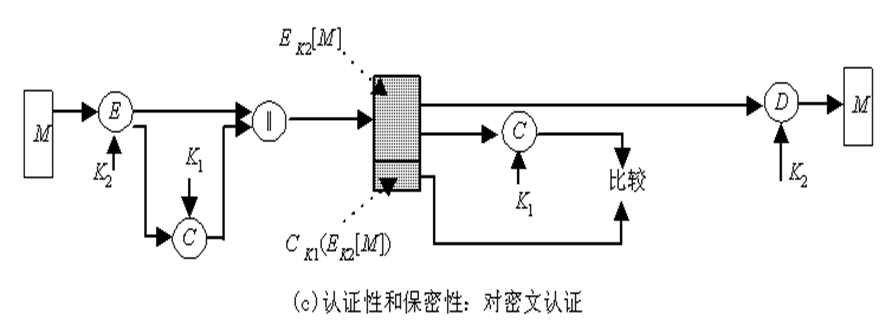#### 5.2.3 数据认证算法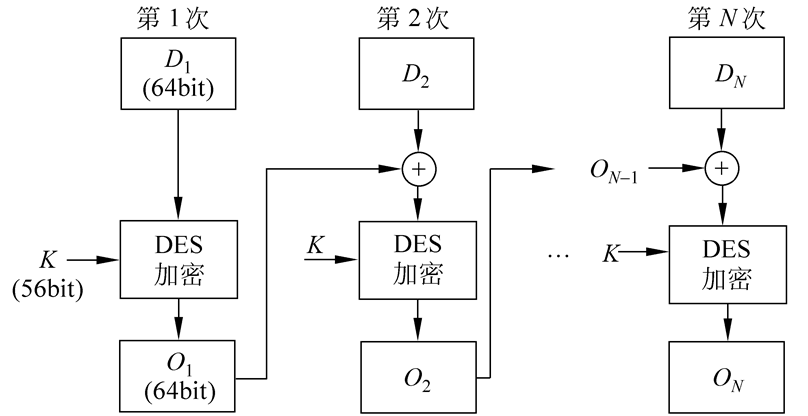其中E为DES加密算法，K为密钥。 数据认证码或者取为$O_N$或者取为$O_N$的最左M个比特，其中16≤M≤64。

### Hash函数

Hash函数是一个将任意长度的消息（message）映射成固定长度消息的函数。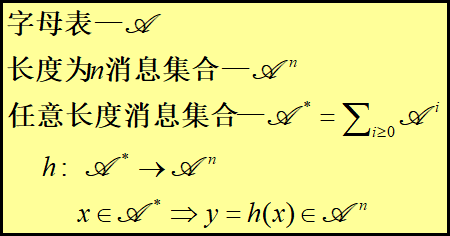将h称为一个Hash函数（hash function），或称为哈希函数、散列函数。对于任何消息x ，将h(x)称为x的Hash值、散列值、消息摘要（message digest）。

h(x)=h(x’)

#### 5.3.2 Hash函数的分类

• 单向Hash函数（one-way） 给定一个Hash值y，如果寻找一个消息x，使得y=h (x)是计算上不可行的，则称h是单向Hash函数.
• 弱抗碰撞Hash函数（weakly collision-free） 任给一个消息x，如果寻找另一个不同的消息x’，使得h(x) =h(x’)是计算上不可行的，则称h是弱抗碰撞Hash函数.
• 强抗碰撞Hash函数 （strongly collision-free） 如果寻找两个不同的消息x和x’，使得h(x)=h(x’)是计算上不可行的，则称h是强抗碰撞Hash函数.

#### 5.3.3 安全Hash函数

• 安全Hash函数h应具有以下性质：
• 对任意的消息x，计算h(x)是容易的；
• h是单向的；
• h是弱抗碰撞的，或是强抗碰撞的。

#### 5.3.4 Hash函数的安全性

• 对Hash函数的攻击是指寻找一对碰撞消息的过程

$(1-\frac{1}{365})(1-\frac{2}{365}...(1-\frac{k-1}{365})$

k人中至少有2人生日相同的概率为：

$p(365,k)=1-(1-\frac{1}{365})(1-\frac{2}{365}...(1-\frac{k-1}{365})$

#### 5.3.4 Hash函数的安全性$(1-\frac{1}{2^n})^R$

$(1-\frac{1}{2^n})^{rR}$

m的变形与M的变形发生碰撞的概率是：

$P(n)=1-(1-\frac{1}{2^n})^{rR}\approx1-e^{-\frac{rR}{2^n}}$

• 用于攻击一类具有特殊结构的Hash函数
• 分析Hash函数运算的中间值相等的概率
• 讨论一类利用加密变换构造的Hash函数

• 用于攻击一类具有特殊结构的Hash函数
• 分析Hash函数运算的中间值相等的概率
• 讨论一类利用加密变换构造的Hash函数
• 攻击方式: 假设攻击者要找出一个假消息M=(M1, M2)，使得M与m是一个碰撞。设m的散列值都为d。攻击者首先产生消息M1的r个变形，消息M2的R个变形.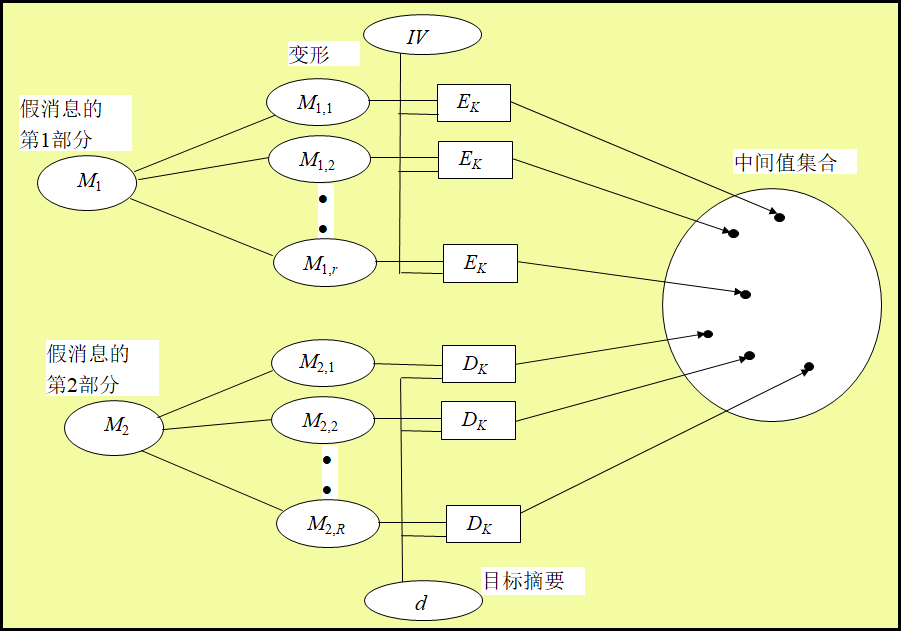$h_1=E_k(m_1,IV)$

$d=h(m)=E_k(m_2,h_1)$

#### 5.3.5 Hash函数的迭代构造法

$f:{0,1}^{m+t}$ →${0,1}^m\space (t\geq 1)$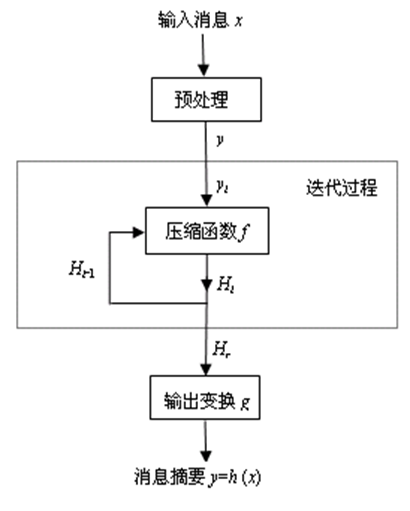• 预处理: 用一个公开算法在消息x右方添加若干比特，得到比特串y，使 得y的长度为t的倍数。即有

$y=x||pad(x)=y_1||y_2||...||y_r$

• 迭代过程: 设$H_0=IV$是一个长度为m的初始比特串，重复使用压缩函数f，依次计算

$Hi= f (H_{i-1}|| y_i) (i=1, 2,…,r)$

• 输出变换: 设g: {0,1}m{0,1}t是一个公开函数，令

$h(x)=g(H_r)$

• 用上述方法构造的Hash函数称为迭代Hash函数。大多数实用Hash函数都是迭代Hash函数
• 在预处理阶段，必须保证变换xy是单射。因为如果预处理变换xy不是单射，则存在xx’使得y=y’，从而h(x)=h(x’)，即能够找到h的碰撞。
• 对于任意无碰撞的压缩函数，都可以使用迭代技术构造一个无碰撞的Hash函数。

### MD5哈希函数

MD5(MD:message digest,消息摘要) 1990年10月, 著名密码学家R. L. Rivest在MIT(Massachusetts Institute of Technology)提出了一种Hash函数,作为RFC 1320 (RFC:互联网研究和开发机构工作记录)公开发表,称为MD4. MD5是MD4的改进版本, 于1992年4月作为RFC 1321公开发表.

• MD5特性
• 直接构造法: 不依赖任何密码系统和假设条件
• 算法简洁
• 计算速度快
• 特别适合32位计算机软件实现
• 倾向于使用低端结构

#### 5.4.1 MD5算法

MD5算法的输入可以是任意长度的消息x，对输入消息按512位的分组为单位进行处理，输出128位的散列值MD(x)。整个算法分为五个步骤。

• 在消息x右边增加若干比特，使其长度与448模512同余。也就是说，填充后的消息长度比512的某个倍数少64位。
• 即使消息本身已经满足上述长度要求，仍然需要进行填充。
• 例如，若消息长为448，则仍需要填充512位使其长度为960位。填充位数在1到512之间。填充比特的第一位是1，其它均为0。

• 经预处理后，原消息长度变为512的倍数
• 设原消息x经预处理后变为消息 $Y=Y0Y_1...Y{L-1}$ 其中$Y_i(i =0,1,...,L-1)$是512比特 在后面的步骤中，将对512比特的分组$Y_i$进行处理

$x_1$= $x$ || 1 || 0 (407个) = $x$ || 800000 00000000 00000000 00000000 00000000 00000000 00000000 00000000 00000000 00000000 00000000 00000000 00000000 =61626364 65800000 00000000 00000000 00000000 00000000 00000000 00000000 00000000 00000000 00000000 00000000 00000000 00000000

$x$=“abcde”=01100001 01100010 01100011 01100100 01100101=(61 62 63 64 65)16, |x|=40=(28)16

• MD5算法的中间结果和最终结果都保存在128位的缓冲区里，缓冲区用4个32位的寄存器表示。
• 4个缓冲区记为A、B、C、D，其初始值为下列32位整数（16进制表示）：

A=67 45 23 01 B=EF CD AB 89 C=98 BA DC FE D=10 32 54 76

• 上述初始值以小端格式存储(字的最低有效字节存储在低地址位置 )为：

• MD5是迭代Hash函数, 其压缩函数为:

$H_{MD5}:{0,1}^{128+512}$ → ${0,1}^{128}$

MD5压缩函数$H_{MD5}$

$H_{MD5}:{0,1}^{128+512}$ → ${0,1}^{128}$

• $H_{MD5}$由四轮处理组成
• 加法是指缓冲区中的4个字与$CV_i$中对应的4个字分别模232相加$H_{MD5}$的四轮处理过程的算法结构相同，每一轮要对缓冲区ABCD进行16次迭代，每次迭代的运算形式为:

$a←b+L^s(a+g(b,c,d)+X[k]+T[i])$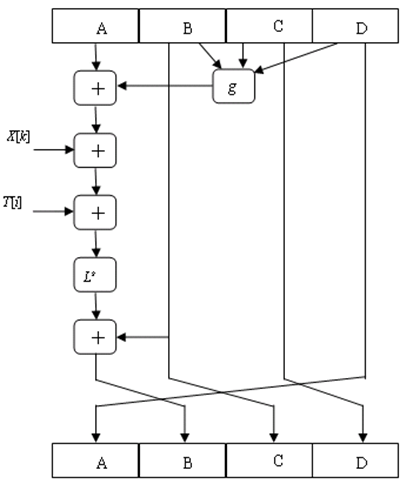• $H_{MD5}$的基本逻辑函数g
• 每一轮使用一个基本逻辑函数g，每个基本逻辑函数的输入是三个32位的字，输出是一个32位的字，它执行位逻辑运算，即输出的第n位是其三个输入的第n位的函数
• 基本逻辑函数g的定义：符号$\wedge$、$\vee$和$-$分别表示逻辑操作AND、OR、NOT和XOR

1 F(b,c,d) $(b\wedge c)\vee (\overline b \wedge d)$
2 G(b,c,d) $(b\wedge d)\vee (c \wedge \overline d)$
3 H(b,c,d) $b\oplus c\oplus d$
4 I(b,c,d) $c\oplus (b\wedge \overline d)$

$H_MD5$的基本逻辑函数g 基本逻辑函数g的真值表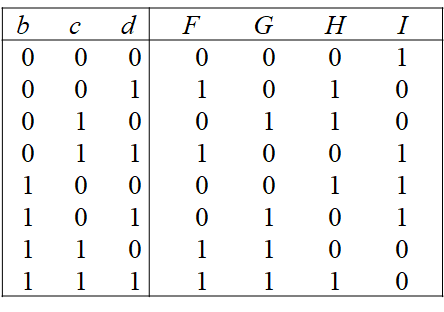• 字组X 把当前处理的512比特的分组Yi依次分成16个32比特的字, 分别记为X[0,1,…,15].
• 在每一轮的16步迭代中, 每一步迭代使用一个字,迭代步数不同使用的字也不相同. 因此, 16步迭代恰好用完16个字。

• 对于不同轮处理过程, 使用16个字的顺序不一样.

• 第一轮中，使用顺序为X[0,1,…,15]。
• 第二轮中使用顺序由下列置换确定: $\rho_2(i)= (1+5i)\space mod\space 16$
• 第三轮中使用顺序由下列置换确定: $\rho_3(i)= (5+3i)\space mod\space 16$
• 第四轮中使用顺序由下列置换确定: $rho_4(i)= 7i\space mod\space 16$
• 例如：第三轮处理过程的第i步迭代使用字 $X[\rho_3(i)]=X[(5+3_i)\space mod\space 16]$ 第8步迭代使用字 $X[\rho_3(8)]=X[(5+3\times 8)]=X=X$

• 常数表T：64个32位常数

• $T[i] =2^{32}\times abs(sin(i))$的整数部分(i=1,2,…,64)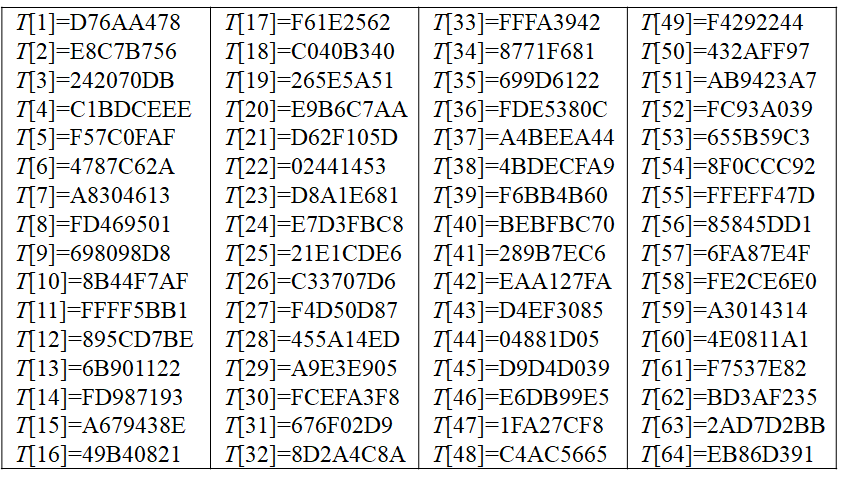• 常数表T的作用是“随机化”32位的输入数据，即消除输入数据的规律性。
• HMD5的第k轮处理过程使用常数表T的元素 $T[16(k-1)+1, 16(k-1)+2,…,16k] (k=1,2,3,4)$ 第k轮的第i次迭代使用元素 $T16(k-1)+ i$

1 7 12 17 22 7 12 17 22 7 12 17 22 7 12 17 22
2 5 9 14 20 5 9 14 20 5 9 14 20 5 9 14 20
3 4 11 16 23 4 11 16 23 4 11 16 23 4 11 16 23
4 6 10 15 21 6 10 15 21 6 10 15 21 6 10 15 21

• $CV_0=IV$
• $CV{i+1}=SUM{32}[CV_i, RF_I(Y_i,RF_H(Y_i,RF_G(Y_i,RF_F(Y_i,CV_i))) )] (i=0,1,…,L-1)$
• $MD=CV_{L-1}$
• $IV$=第三步定义的缓冲区ABCD的初值
• L =消息经第一步和第二步处理后分组的个数
• $Y_i$ =消息的第i个512位分组
• $RF_u$ =使用基本逻辑函数u的轮函数
• $SUM_{32}$=对输入字的模232相加
• MD =散列值

### 5.4.2 MD5的安全性

• Rivest猜测，MD5可能是128位Hash函数中强度最大的。
• 目前，对MD5的攻击已取得以下结果：

• T. Berson（1992）已经证明，对单轮的MD5算法，利用差分密码分析，可以在合理的时间内找出散列值相同的两条消息。这一结果对MD5四轮运算的每一轮都成立。但是，目前尚不能将这种攻击推广到具有四轮运算的MD5上.
• B. Boer和A. Bosselaers（1993）说明了如何找到消息分组和MD5两个不同的初始值,使它们产生相同的输出. 也就是说, 对一个512位的分组, MD5压缩函数对缓冲区ABCD的不同值产生相同的输出,这种情况称为伪碰撞（pseudo-collision）.目前尚不能用该方法成功攻击MD5算法.
• 目前，对MD5的攻击已取得以下结果(续)：

• H. Dobbertin（1996）找到了MD5无初始值的碰撞(pseudo-collision).给定一个512位的分组,可以找到另一个512位的分组,对于选择的初始值IV0,它们的MD5运算结果相同. 到目前为止, 尚不能用这种方法对使用MD5初始值IV的整个消息进行攻击.
• 我国山东大学王小云教授（2004）提出的攻击对MD5最具威胁。对于MD5的初始值IV，王小云找到了许多512位的分组对，它们的MD5值相同.
• 国际密码学家Lenstra利用王小云等提供的MD5碰撞，伪造了符合X.509标准的数字证书.
• MD5算法抗密码分析能力较弱,对MD5的生日攻击所需代价为264数量级. 所以, 必须设计新的Hash算法, 使其与MD5相比具有更长的散列值和更高的安全性.

### 安全哈希算法SHA-1

#### 5.5.1 SHA-1算法步骤

SHA-1处理消息的过程与MD5类似，对输入消息按512位的分组为单位进行处理，整个算法分为五个步骤

• 步骤1与步骤2一起称为消息的预处理

SHA-1算法的中间结果和最终结果保存在160位的缓冲区里，缓冲区用5个32位的寄存器表示。5个缓冲区记为A、B、C、D、E，其初始值为下列32位整数（16进制表示）：

A=67 45 23 01 B=EF CD AB 89 C=98 BA DC FE D=10 32 54 76 E=C3 D2 E1 F0

• 步骤4: 以512位的分组（16个字）为单位处理消息 SHA-1是迭代Hash函数，其压缩函数为：

$H_{SHA}:{0,1}^{160+512}→{0,1}^{160}$

• 步骤4是SHA-1算法的主循环，它以512比特作为分组，重复应用压缩函数HSHA，从消息Y的第一个分组$Y1$开始，依次对每个分组$Y_i$进行压缩，直至最后分组Y{L-1}，然后输出消息x的Hash值。 SHA-1循环次数等于消息Y中512比特分组的数目L。

SHA-1的压缩函数$H_{SHA}$

• 由四轮处理组成
• 加法是模232相加
• 压缩函数HSHA的四轮处理过程的算法结构相同，每一轮要对缓冲区ABCDE进行20次迭代，每次迭代的运算形式为

$A,B,C,D,E\space ←\space (E+f(B,C,D)+L^5(A)+W_t+K_t),A,L^{30}(B),C,D$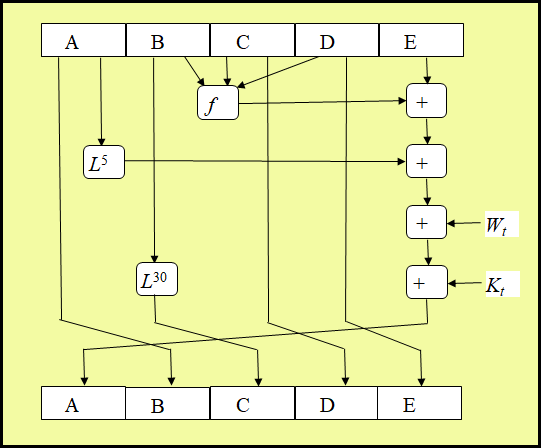• 基本逻辑函数f
• 每一轮使用一个基本逻辑函数f，每个基本逻辑函数的输入是三个32位的字，输出是一个32位的字，它执行位逻辑运算，即输出的第n位是其三个输入第n位的函数。

1 $f_1(B,C,D)$ $(B\wedge C)\vee (\overline B \wedge D)$
2 $f_2(B,C,D)$ $B\oplus C \oplus D$
3 $f_3(B,C,D)$ $(B\wedge C)\vee (B \wedge D)\vee (C\wedge D)$
4 $f_4(B,C,D)$ $B\oplus C \oplus D$
• 基本逻辑函数f
• 基本逻辑函数f的真值表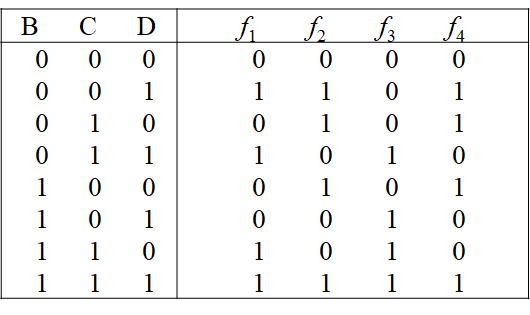• 字组$W_t$

• t（0≤t≤79）代表迭代步数，依次表示第一、二、三、四轮处理过程进行的迭代次序
• $Wt(0≤t≤79)$是32比特的字，它的前面16个字$W_0,W_1,…,W{15}$依次取自当前输入分组$Y_i$，其余字为

$Wt=L^1(W{t-16}\oplus W{t-14}\oplus W{t-3})\space (t=16,17,...79)$

$0\leq t \leq 19$ 5A827999 [$2^{30}\times \sqrt{2}$]
$20\leq t \leq 39$ 6ED9EBA1 [$2^{30}\times \sqrt{3}$]
$40\leq t \leq 59$ 8F1BBCDC [$2^{30}\times \sqrt{5}$]
$60\leq t \leq 79$ CA62C1D6 [$2^{30}\times \sqrt{10}$]

• 第L个分组处理后的输出值即是消息x的散列值MD(x)
• SHA-1的处理过程归纳如下：
• $CV_0=IV$
• $CV{i+1}=SUM{32}(CV_i, ABCDE_i ) (i=0,1,…, L-1)$
• $MD= CV_{L-1}$
• 其中：
• IV =第三步定义的缓冲区ABCDE的初值
• $ABCDE_i$ =处理第i个消息分组时最后一轮的输出
• L =消息经第一步和第二步处理后分组的个数
• SUM_{32}=对输入字的模$2^{32}$相加
• MD =散列值

#### 5.5.2 SHA-1和MD5的比较

• SHA-1与MD5的算法类似，所以它们的性质极为相似
• 抗穷举攻击的能力
• SHA1抗穷举攻击的能力比MD5强
• 用穷举攻击方法产生具有给定散列值的消息
• MD5需要的代价为2128数量级
• SHA1需要的代价为2160数量级
• 用穷举攻击方法产生两个具有相同散列值的消息
• MD5需要的代价为264数量级
• SHA1需要的代价为280数量级
• 抗密码分析的能力

• MD5算法抗密码分析的能力较弱
• SHA1算法抗密码分析的能力似乎并不弱
• 速度 SHA-1执行的速度比MD5的速度慢得多

• 简洁性 SHA-1和MD5两种算法都易于描述和实现，不需要使用大的程序和置换表
• 数据的存储方式 MD5使用little-endian方式，SHA-1使用big-endian方式。这两种方式没有本质的差异

### 基于分组密码与离散对数的哈希函数

Hash函数的间接构造法

• 利用已有的密码算法构造Hash函数
• 如果密码算法是安全的，那么利用它所构造的Hash函数也是安全的

#### 5.6.1 利用分组密码算法构造Hash函数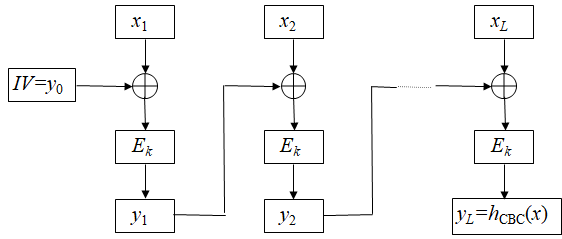• 首先选取一个初始值: y0 =IVGF(2)n，
• 然后依次计算: $yi=x_i\oplus E_k(y{i-1})(1\leq i\leq L)$ 最后定义Hash值为: $h_{CFB}(x)=y_L$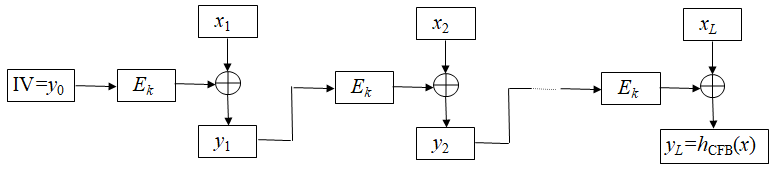#### 5.6.2 基于离散对数问题的Hash函数

• 基于一些困难数学问题，诸如离散对数问题、因子分解问题、背包问题等可以构造出一些Hash函数，这些Hash函数的安全性依赖于对应数学问题的困难性
• Chaum、Heijst和Pfitzmann（1992年）提出的基于离散对数问题构造的Hash函数
• 运行速度不是很快
• 可以证明是安全的.
• Chaum-Heijst-Pfitzmann Hash函数的构造 设p是一个大素数，$q=(p-1)/2$是一个素数，$\alpha$和$\beta$是$Zp$的两个本原元。假设离散对数$log{\alpha}\beta$是计算上不可行的。定义Hash函数$h$为： $h:Z_p\times Z_p → Z_p^{*}$ $h(x_1,x_2)=\alpha^{x_1}\times \beta^{x_2}\space mod \space p$

Chaum-Heijst-Pfitzmann Hash函数是强抗碰撞的，用反证法，如果Hash函数h有一对碰撞，那么可以证明离散对数$log^{\alpha}\beta$能被有效计算.

• 情况1：d =1 此时$x_4-x_2$关于模p-1有逆，设$y=(x_4-x_2)^{-1}\space mod\space(p-1)$，则存在整数k，使得$(x_4-x_2)y=1+(p-1)k$，则有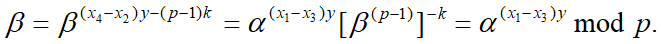因此，可计算离散对数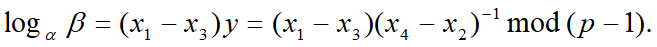• 情况2：d =2 因为$p-1=2q$,且q是奇数,所以$gcd(x_4-x_2, q)=1$。设$y=(x_4-x_2)^{-1}\space mod\space q$，则存在整数k，使得 $(x_4-x_2) y=1+qk$, 有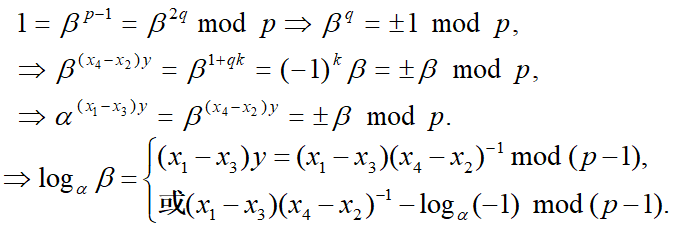• 情况3：d = q 因为 0≤x2≤q-1, 0≤x4≤q-1 $\rightarrow -(q-1)≤x4-x2≤q-1 \rightarrow gcd(x4-x2, q-1)= q$不成立。 情况3不存在.

• 情况4：d =p-1 这种情况只有在$x_2=x_4$时才可能发生。这样就有 $\alpha^{x-1}=\alpha^{x_3}\space mod\space p$ 所以$x_1=x_3,(x_1, x_2)= (x_3, x_4)$，与已知矛盾! 即情况4也不存在。